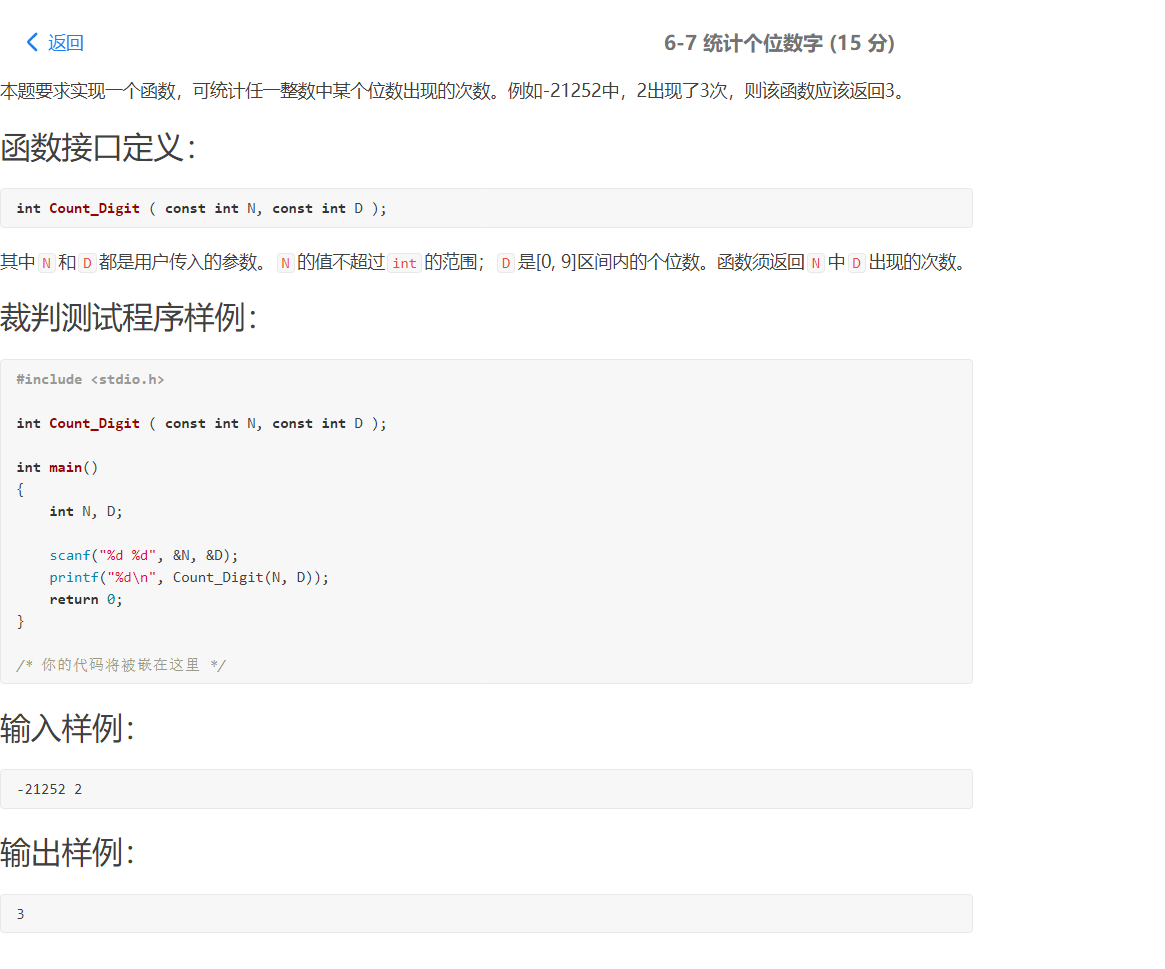• @[pta题目：数字统计] ##问题描述： CAISZ有个女儿，已上幼儿园，在幼儿园中她学会了数数，但还不会做统计。现给定一个数字串，长度小于100，统计0，1，2……9 的个数。 好心的你帮帮她吧。 输入格式: 输入多组数字...
@[pta题目：数字统计]
##问题描述：
CAISZ有个女儿，已上幼儿园，在幼儿园中她学会了数数，但还不会做统计。现给定一个数字串，长度小于100，统计0，1，2……9 的个数。 好心的你帮帮她吧。
输入格式: 输入多组数字串，长度小于100；
输出格式: 数字串中的各个数字的个数，数字统计按照0，1，2，3。。9的顺序输出
输入样例: 在这里给出一组输入。例如：
11122233444445078 输出样例: 在这里给出相应的输出。例如：
0:1 1:3 2:3 3:2 4:5 5:1 6:0 7:1 8:1 9:0 。
本题首先用c语言写😊，浅显易懂，适合初学者学习研究
代码如下：
#include<stdio.h>//编译预处理命令
#include<string.h>//库函数的头文件
int main()
{
int i,j,b,n0,n1,n2,n3,n4,n5,n6,n7,n8,n9,k;
n0=n1=n2=n3=n4=n5=n6=n7=n8=n9=0;
char a;//此处定义了一个长度为一百的数组
while(scanf("%s",a)!=EOF)//注意以%s输入
{
j=strlen(a);//统计数字字符个数
for(i=0;i<j;i++)
{
switch (a[i])//开关语句
{
case '0':
n0++;break;
case '1':
n1++;break;
case '2':
n2++;break;
case '3':
n3++;break;
case '4':
n4++;break;
case '5':
n5++;break;
case '6':
n6++;break;
case '7':
n7++;break;
case '8':
n8++;break;
case '9':
n9++;break;

}

}
printf("0:%d\n",n0);
printf("1:%d\n",n1);
printf("2:%d\n",n2);
printf("3:%d\n",n3);
printf("4:%d\n",n4);
printf("5:%d\n",n5);
printf("6:%d\n",n6);
printf("7:%d\n",n7);
printf("8:%d\n",n8);
printf("9:%d\n",n9);
n0=n1=n2=n3=n4=n5=n6=n7=n8=n9=0;
}
return 0;
}

看上去更高级一点的代码：
#include<bits/stdc++.h>
using namespace std;
int main()
{
int i,j,k;
int sum={0};
char a;
char b={'0','1','2','3','4','5','6','7','8','9'};
scanf("%s",a);
j=strlen(a);
for(i=0;i<j;i++)
{
for(k=0;k<10;k++)
{
if(a[i]==b[k])
sum[k]++;
}
}
for(i=0;i<10;i++)
cout<<i<<':'<<sum[i]<<endl;
return 0;
}

代码的原理比较简单，注意题目要求输入多组数字串运用while(scanf("%s",a)!=EOF)，注意以%s输入（因为字符串为纯数字,无空格，可用%s)，之后运用开关语句即可。
展开全文c语言 字符串
• int Count_Digit ( const int N, const int D ){ int b=N,count=0; if(b<0){ b=(-1)*b; } if(b==0&&D==0){ count=1; } while(b>0){ if(b%10==D){ count++;...用b代替N的值进.int Count_Digit ( const int N, const int D ){
int b=N,count=0;
if(b<0){
b=(-1)*b;
}
if(b==0&&D==0){
count=1;
}
while(b>0){
if(b%10==D){
count++;
}
b=b/10;
}
return count;
}

注：const修饰的数据类型是指常类型，具有不可变性； 所以int b=N；用b代替N的值进行操作。
展开全文c语言
• 接收输入的一行字符，统计出字符串中包含数字的个数。 输入格式: 输入一行任意的字符串，可以包含包含数字、字母和特殊字符，也可以不包括 输出格式: 输出输入字符串中数字的个数 输入样例1: 在这里给出一组输入。...
接收输入的一行字符，统计出字符串中包含数字的个数。
输入格式: 输入一行任意的字符串，可以包含包含数字、字母和特殊字符，也可以不包括
输出格式: 输出输入字符串中数字的个数
输入样例1: 在这里给出一组输入。例如：
hello
#结尾无空行

输出样例1: 在这里给出相应的输出。例如：
0
#结尾无空行

输入样例2: 在这里给出一组输入。例如：
a123b*4_5
#结尾无空行

输出样例2: 在这里给出相应的输出。例如：
5
#结尾无空行

代码：
str= input()
sum= 0

for key in str:
if key >= '0' and key <= '9':
sum += 1

print(sum)


展开全文python
• ﻿﻿ int CountDigit( int number, int digit ){  int count=0;  if(number  number=-number;  while(number){  if((number%10)==digit)  count++;  number=number/10;  } ...//全0

﻿﻿

int CountDigit( int number, int digit ){  int count=0;  if(number<0)   number=-number;   while(number){   if((number%10)==digit)    count++;   number=number/10;  }    return count;
}
//全0的情况未考虑
//解决方法：while换成do while

展开全文• 6-1 统计各位数字之和是5的数 (10 分) 本题要求实现两个函数：一个函数判断给定正整数的各位数字之和是否等于5；另一个函数统计给定区间内有多少个满足上述要求的整数，并计算这些整数的和。 函数接口定义： int is...
• 本题要求编写程序读入N个学生的百分制成绩，统计五分制成绩的分布。百分制成绩到五分制成绩的转换规则：大于等于90分为A；小于90且大于等于80为B；小于80且大于等于70为C；小于70且大于等于60为D；小于60为E。输入...
• 【Python】L1-003 个位数统计-PTA团体程序设计天梯赛 给定一个 k 位整数，请编写程序统计每种不同的个位数字出现的次数。例如：给定 N=100311，则有 2 个 0，3 个 1，和 1 个 3。 输入格式： 每个输入包含 1 个测试...Python
• 题目链接 题目代码： #include <stdio.h> int Count_Digit ( const int N, const int D ); int main() { int N, D; scanf("%d %d", &N, &D); printf("%d\n", Count_...注意：要考虑到N的...
•c 桶排序
• 本题要求实现两个函数：一个函数判断给定正整数的各位数字之和是否等于5；另一个函数统计给定区间内有多少个满足上述要求的整数，并计算这些整数的和。 int is( int number ); void count_sum( int a, int b ); ...
• 本题要求实现一个统计整数中指定数字的个数的简单函数。 CountDigit(number,digit ) 其中number是整数，digit为[1, 9]区间内的整数。函数CountDigit应返回number中digit出现的次数。 函数接口定义： CountDigit...python
• 本题要求实现一个函数，可统计任一整数中某个位数出现的次数。例如-21252中，2出现了3次，则该函数应该返回3。 函数接口定义： int Count_Digit ( const int N, const int D ); 其中N和D都是用户传入的参数。N...C语言
• 本题要求实现一个函数，可统计任一整数中某个位数出现的次数。例如-21252中，2出现了3次，则该函数应该返回3。 函数接口定义： int Count_Digit ( const int N, const int D ); 其中N和D都是用户传入的参数。N的值...c++
• [PTA]实验5-8 使用函数统计指定数字的个数 本题要求实现一个统计整数中指定数字的个数的简单函数。 函数接口定义： int CountDigit( int number, int digit ); 其中number是不超过长整型的整数，digit为[0, 9]区间...C 模运算
• [PTA]习题5-5 使用函数统计指定数字的个数 本题要求实现一个统计整数中指定数字的个数的简单函数。 函数接口定义： int CountDigit( int number, int digit ); 其中number是不超过长整型的整数，digit为[0, 9]区间内...c语言...